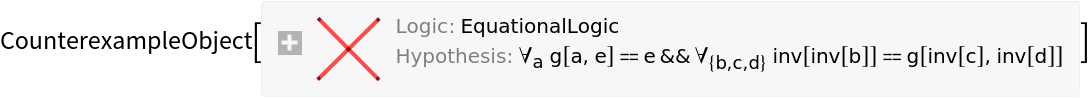#Function Repository Resource:

# FindEquationalCounterexample

Try to find a counterexample to an equational hypothesis in a given system of axioms

Contributed by: Jonathan Gorard
 ResourceFunction["FindEquationalCounterexample"][hyp,axms] tries to find an equational counterexample to the symbolic hypotheses hyp using the axioms axms.

## Details and Options

If ResourceFunction["FindEquationalCounterexample"][hyp,axms] succeeds in finding a counterexample to the hypotheses hyp given the axioms axms, then it returns a CounterexampleObject expression.
Axioms and hypotheses must be given in equational form.
The inputs hyp and axms can be any logical combination of terms of the form:
 expr1⩵expr2 assertion of equality ForAll [ vars , stmt ] assertion that stmt is true for all values of vars
The expri can be arbitrary symbolic expressions, with heads representing formal operators, functions etc.
ResourceFunction["FindEquationalCounterexample"][expr1&&expr2&&,axm1&&axm2&&] will treat each expri as a separate hypothesis and each axmi as a separate axiom.
ResourceFunction["FindEquationalCounterexample"][{expr1,expr2,},{axm1,axm2,}] is equivalent to ResourceFunction["FindEquationalCounterexample"][expr1&&expr2&&,axm1&&axm2&&].
ResourceFunction["FindEquationalCounterexample"] uses the back end of FindEquationalProof to find an appropriate counterexample to the hypothesis.

## Examples

### Basic Examples (2)

Find a counterexample to an elementary hypothesis in group theory:

 In:=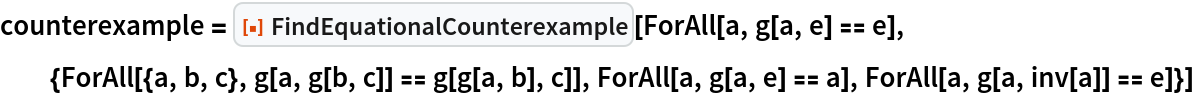Out=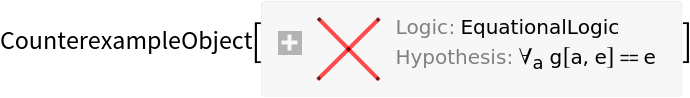Show the counterexample association:

 In:=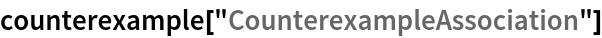Out=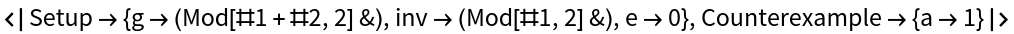Generate a function to verify that this is a valid counterexample to the hypothesis:

 In:=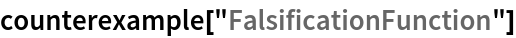Out=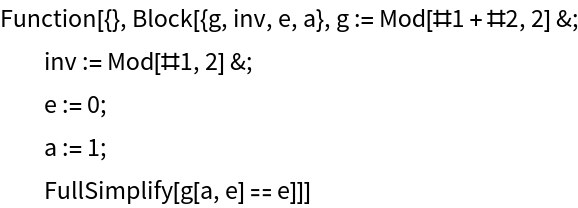In:=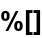Out=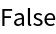Generate a function to verify that this counterexample is consistent with the underlying axioms:

 In:=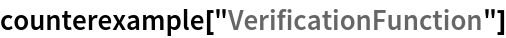Out=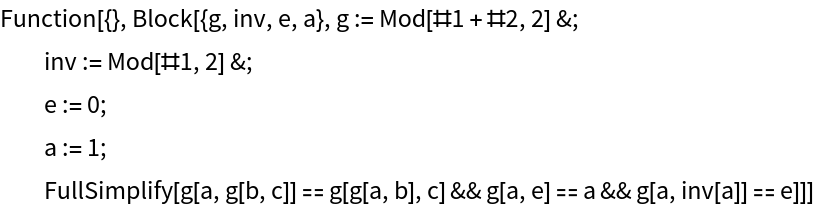In:=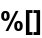Out=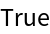Find a counterexample to a more sophisticated hypothesis in group theory:

 In:=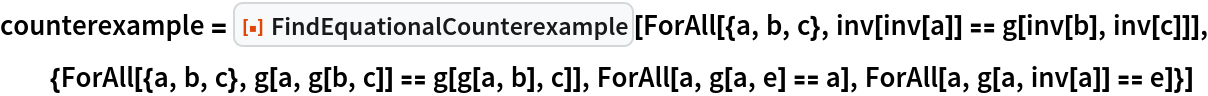Out=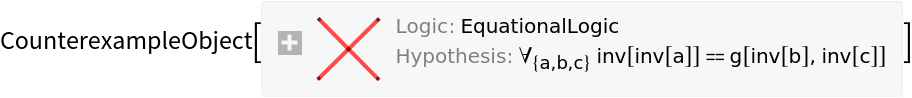Show the counterexample association:

 In:=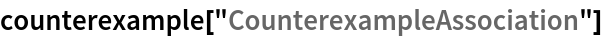Out=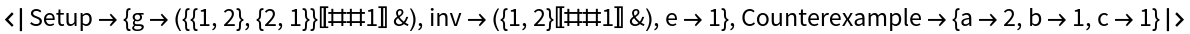Generate a function to verify that this is a valid counterexample to the hypothesis:

 In:=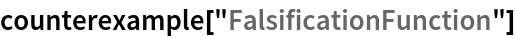Out=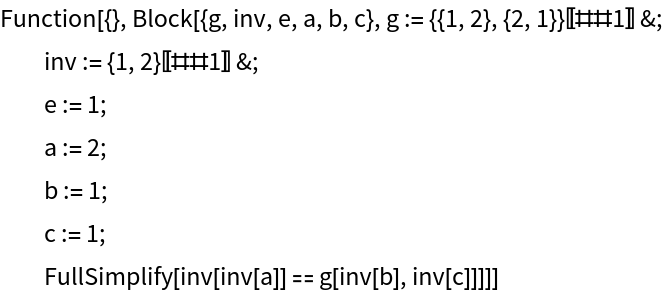In:=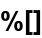Out=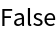Generate a function to verify that this counterexample is consistent with the underlying axioms:

 In:=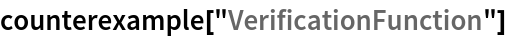Out=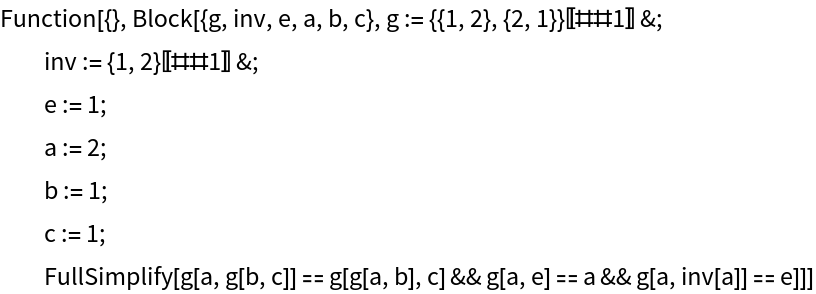In:=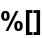Out=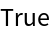### Scope (5)

#### Axiom Systems (3)

FindEquationalCounterexample supports hypotheses formulated in any equational axiom system, including Wolfram's shortest possible axiom for Boolean algebra (finding a counterexample to the hypothesis that the Nand operator is associative):

 In:=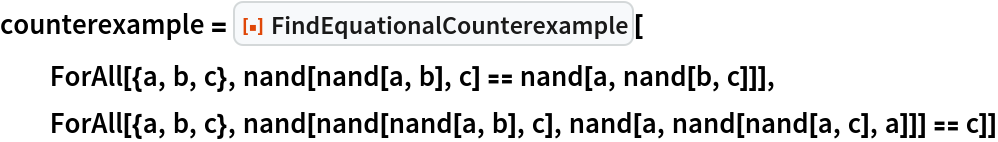Out=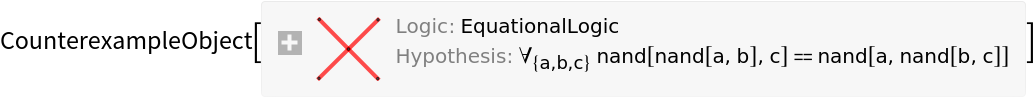Show the counterexample association:

 In:=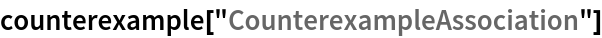Out=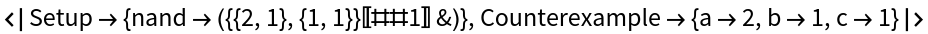Generate a function to verify that this is a valid counterexample to the hypothesis:

 In:=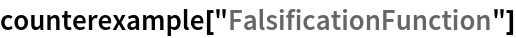Out=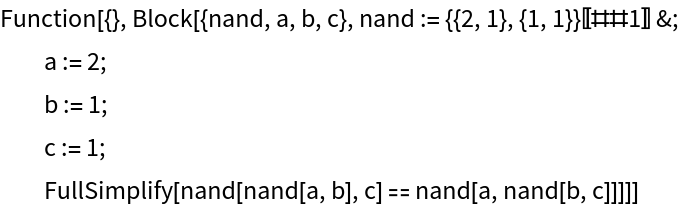In:=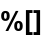Out=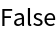Generate a function to verify that this counterexample is consistent with the underlying axioms:

 In:=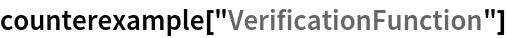Out=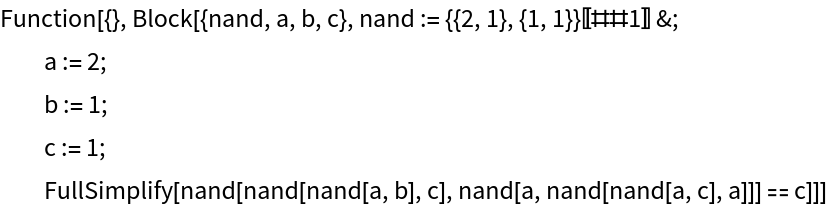In:=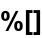Out=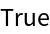Ring theory (finding a counterexample to the hypothesis that every ring is commutative):

 In:=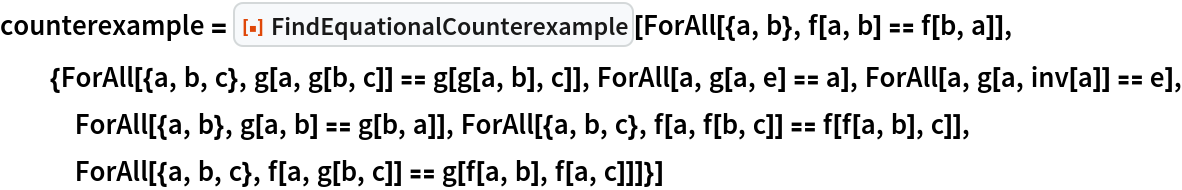Out=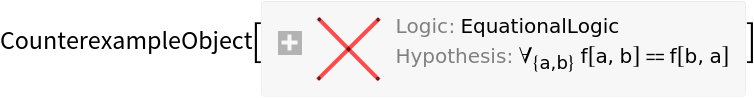Show the counterexample association:

 In:=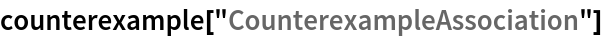Out=Generate a function to verify that this is a valid counterexample to the hypothesis:

 In:=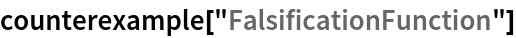Out=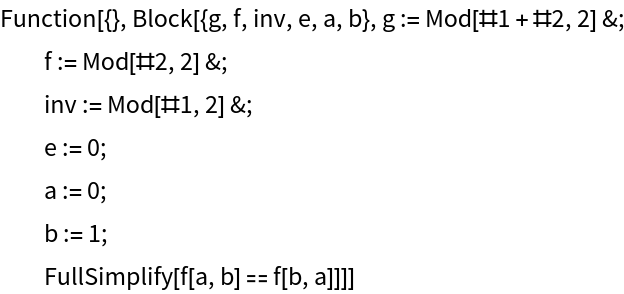In:=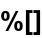Out=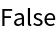Generate a function to verify that this counterexample is consistent with the underlying axioms:

 In:=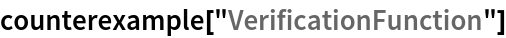Out=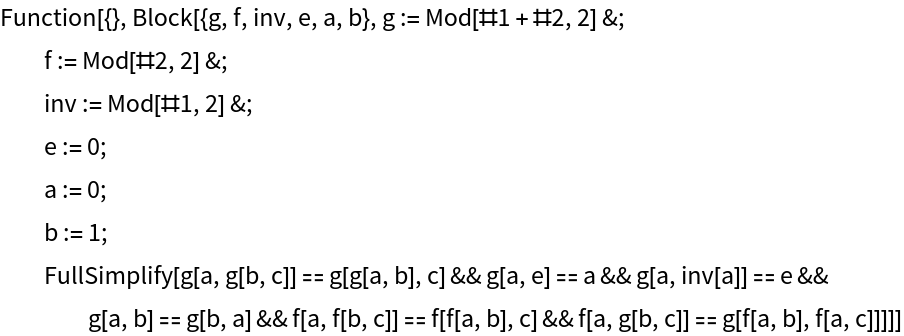In:=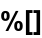Out=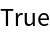Equational Presburger arithmetic (finding a counterexample to the hypothesis that every integer is equal to its negation):

 In:=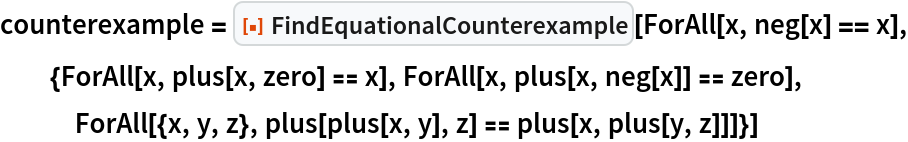Out=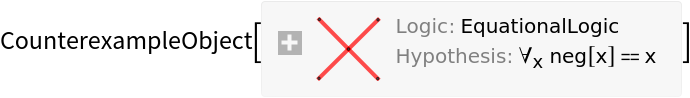Show the counterexample association:

 In:=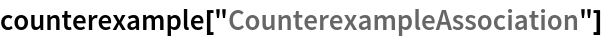Out=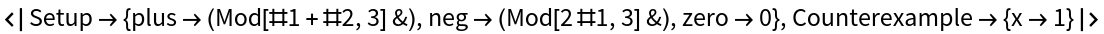Generate a function to verify that this is a valid counterexample to the hypothesis:

 In:=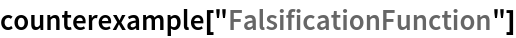Out=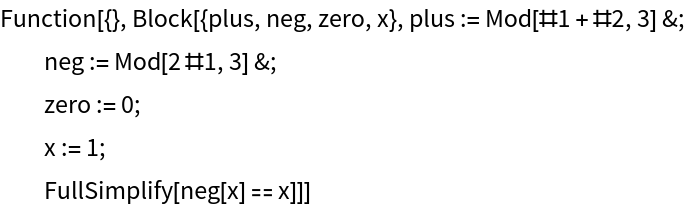In:=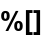Out=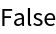Generate a function to verify that this counterexample is consistent with the underlying axioms:

 In:=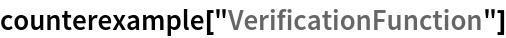Out=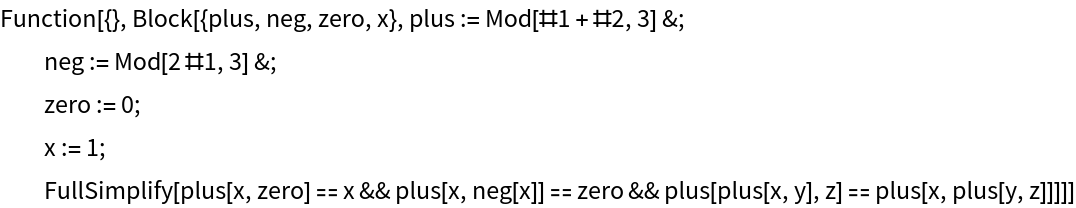In:=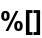Out=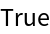#### Hypothesis and Axiom Specification (2)

Find a counterexample from a list of axioms:

 In:=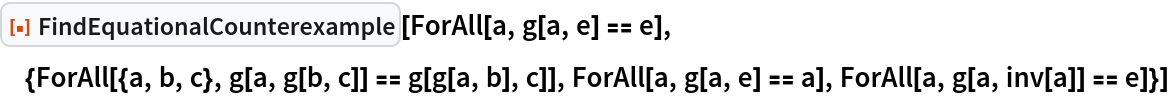Out=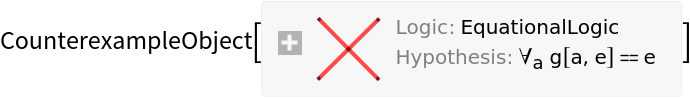Express the axioms as a conjunction:

 In:=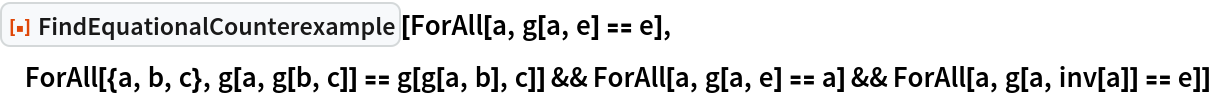Out=Find a counterexample to a list of hypotheses:

 In:=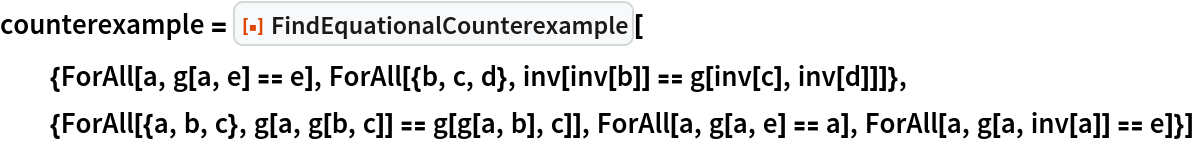Out=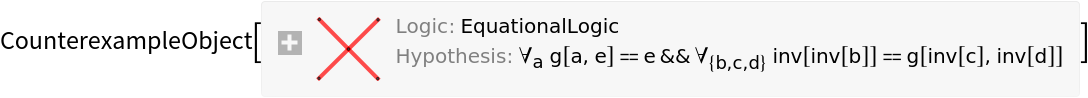In:=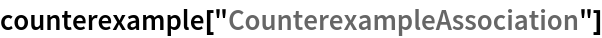Out=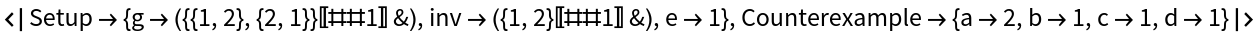Express the hypotheses as a conjunction:

 In:=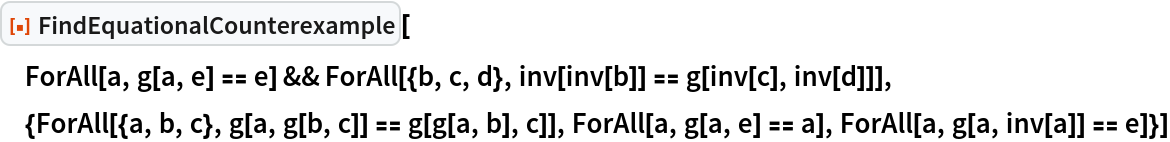Out=